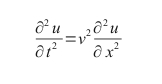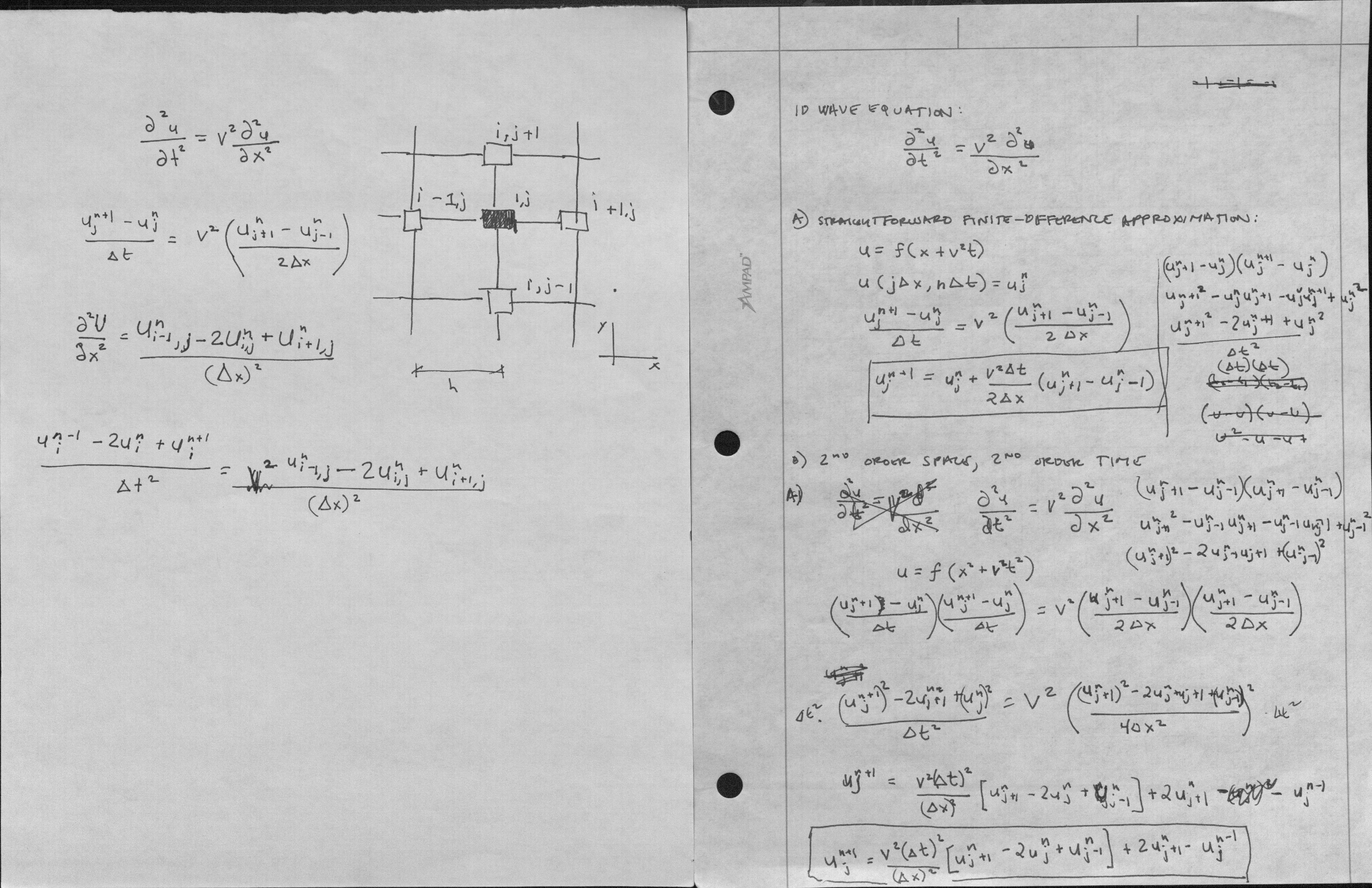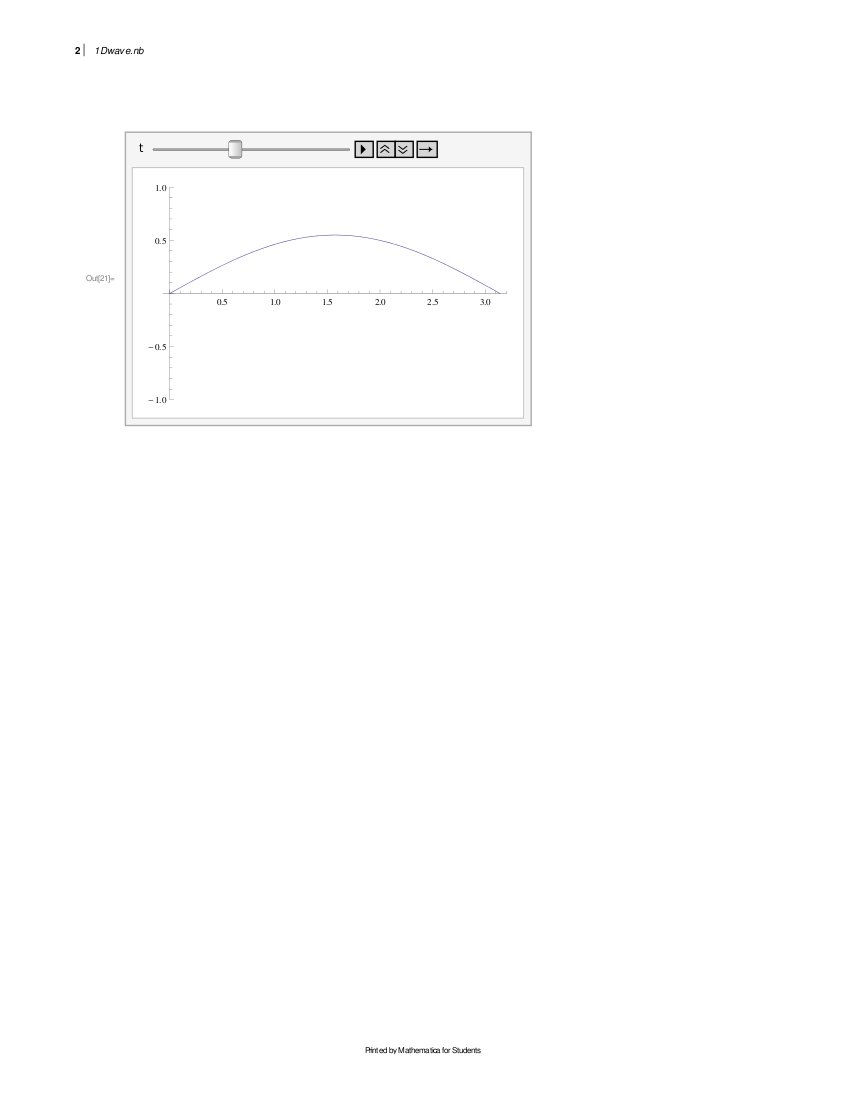The Nature of Mathematical Modeling - Rachelle Villalon

Finite Differences: Partial Differential Equations

 Consider the 1D wave equation:Like most of these problems, I like to visualize what's going on and arrive at an intuitive interpretation of an equation, in this case, the wave equation:Write down the straightforward finite-difference approximation. I've made more than one attempt at writing down the straightforward finite-difference approximation (right image) for the 1D wave equation.The answer made more sense to me after the second try (seen on the left image):What order approximation is this in time and in space? Second order x and t. Use the von Neumann stability crtierion to find the mode amplitudes:Numerically solve the wave equation. Using Mathematica:.textContainer { font-family: "HelveticaNeue-Light", "Helvetica Neue Light", "Helvetica Neue", Helvetica, Arial, "Lucida Grande", sans-serif; font-weight: 300; font-size: 15px; }